Normal Force Card Sort - Questions

The Normal Force Card Sort Concept Builder is comprised of 12 questions that involve the same task. Each question includes a card depicting a situation involving an object in contact with a scale that reads the normal force between the scale top and the object. Students must sort the cards into categories based upon the relative size of the normal force - either equal to the object weight, greater than the object weight, or less than the object weight ... or not enough information to decide.

There are three levels of difficulty by which the student can progress through the Concept Builder. Those three difficulty levels are differentiated as follows:

• Apprentice Difficulty Level: Questions 1-6 ... what we perceive to be the easiest situations.
• Masters Difficulty Level: Questions 7-12 ...  what we perceive to be the most difficult situations.
• Wizard Difficulty Level: Questions 1-12 ... all 12 situations from the two easier difficulty levels.

The questions are shown below. Teachers are encouraged to view the questions in order to judge which level of difficulty is most appropriate for their classes. We recommend providing students two or more options. For instance, it would be profitable for a student to complete the Concept Builder at the Apprentice Level in order to gain some confidence and then to be challenged to complete the activity at the Masters Level.

The Physics Classroom grants teachers and other users the right to print these questions for private use. Users are also granted the right to copy the text and modify it for their own use. However, this document should not be uploaded to other servers for distribution to and/or display by others. The Physics Classroom website should remain the only website or server from which the document is distributed or displayed. We also provide a PDF that teachers can use under the same conditions. We have included a link to the PDF near the bottom of this page.

Normal Force Card Sort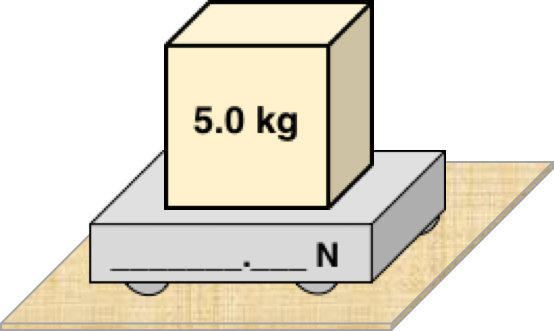Question 1
Consider the following situation and categorize it according to the relative strength of the normal force (or scale reading). Category options include:
1. The normal force is equal to the weight of the object.
2. The normal force is greater than (>)the weight of the object.
3. The normal force is less than (<) the weight of the object.
4. There is not enough information to decide.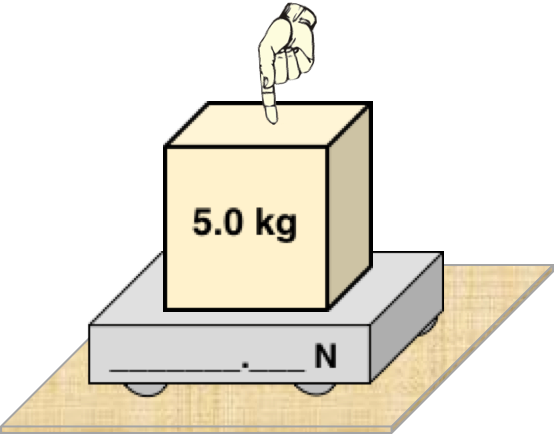Question 2
Consider the following situation and categorize it according to the relative strength of the normal force (or scale reading). Category options include:
1. The normal force is equal to the weight of the object.
2. The normal force is greater than (>)the weight of the object.
3. The normal force is less than (<) the weight of the object.
4. There is not enough information to decide.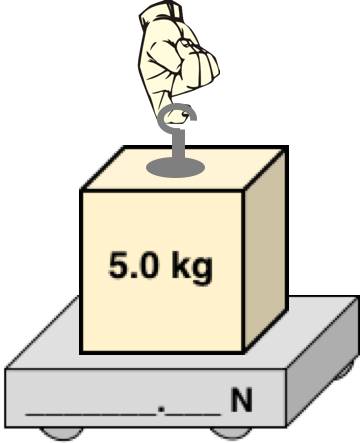Question 3
Consider the following situation and categorize it according to the relative strength of the normal force (or scale reading). Category options include:
1. The normal force is equal to the weight of the object.
2. The normal force is greater than (>)the weight of the object.
3. The normal force is less than (<) the weight of the object.
4. There is not enough information to decide.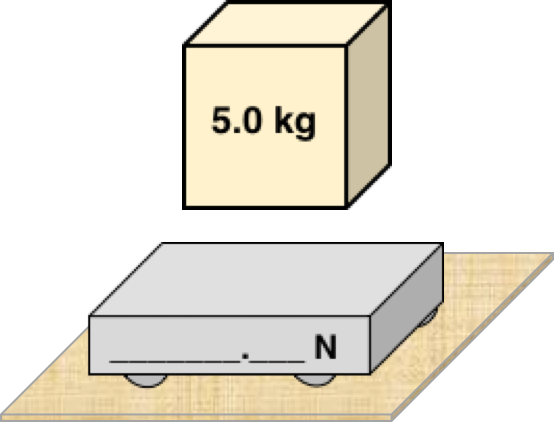Question 4
Consider the following situation and categorize it according to the relative strength of the normal force (or scale reading). Category options include:
1. The normal force is equal to the weight of the object.
2. The normal force is greater than (>)the weight of the object.
3. The normal force is less than (<) the weight of the object.
4. There is not enough information to decide.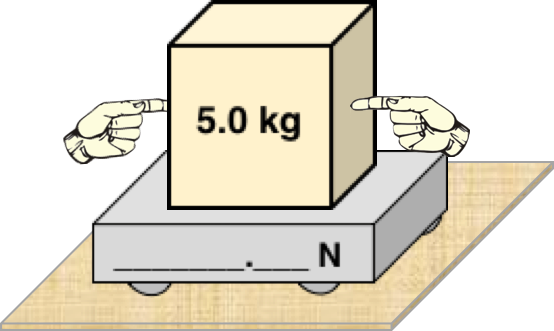Question 5
Consider the following situation and categorize it according to the relative strength of the normal force (or scale reading). Category options include:
1. The normal force is equal to the weight of the object.
2. The normal force is greater than (>)the weight of the object.
3. The normal force is less than (<) the weight of the object.
4. There is not enough information to decide.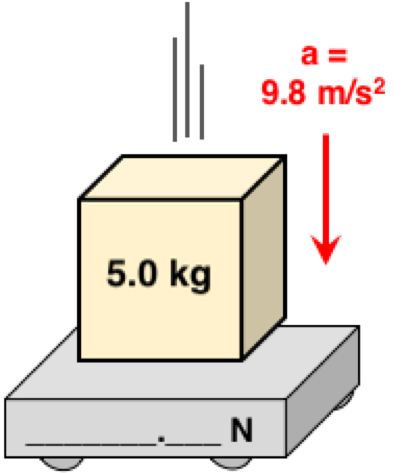Question 6
Consider the following situation and categorize it according to the relative strength of the normal force (or scale reading). Category options include:
1. The normal force is equal to the weight of the object.
2. The normal force is greater than (>)the weight of the object.
3. The normal force is less than (<) the weight of the object.
4. There is not enough information to decide.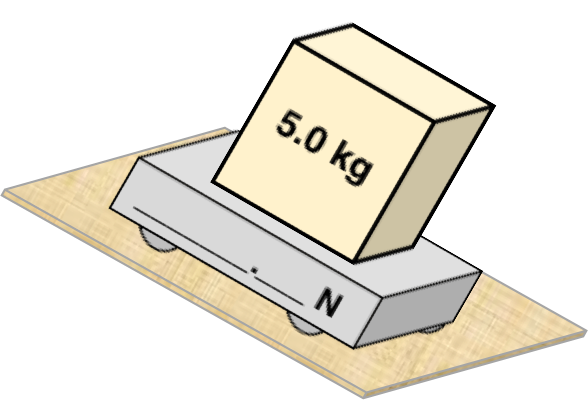Question 7
Consider the following situation and categorize it according to the relative strength of the normal force (or scale reading). Category options include:
1. The normal force is equal to the weight of the object.
2. The normal force is greater than (>)the weight of the object.
3. The normal force is less than (<) the weight of the object.
4. There is not enough information to decide.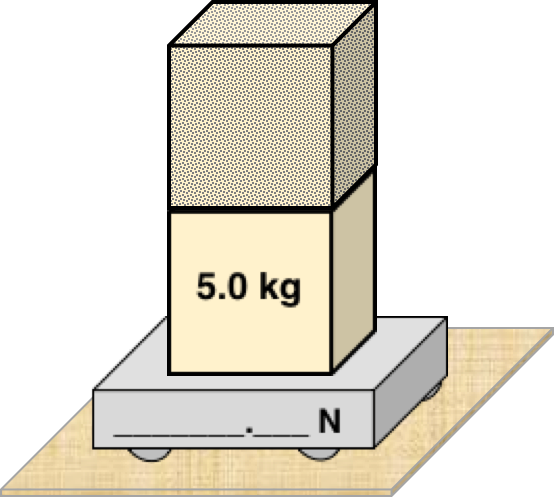Question 8
Consider the following situation and categorize it according to the relative strength of the normal force (or scale reading). Category options include:
1. The normal force is equal to the weight of the object.
2. The normal force is greater than (>)the weight of the object.
3. The normal force is less than (<) the weight of the object.
4. There is not enough information to decide.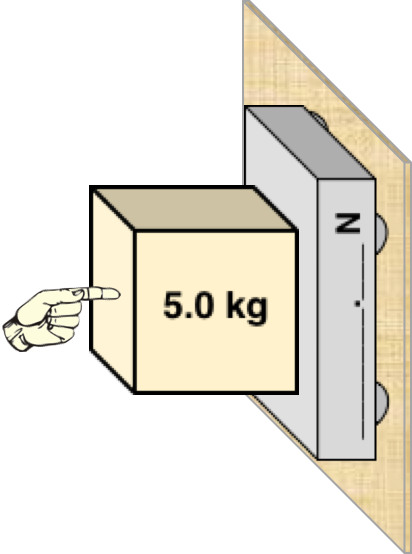Question 9
Consider the following situation and categorize it according to the relative strength of the normal force (or scale reading). Category options include:
1. The normal force is equal to the weight of the object.
2. The normal force is greater than (>)the weight of the object.
3. The normal force is less than (<) the weight of the object.
4. There is not enough information to decide.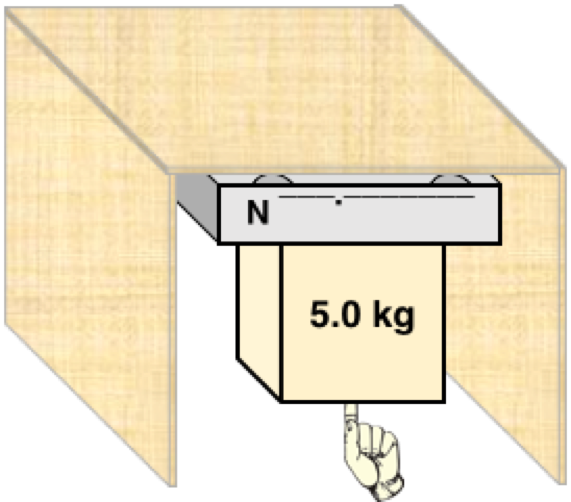Question 10
Consider the following situation and categorize it according to the relative strength of the normal force (or scale reading). Category options include:
1. The normal force is equal to the weight of the object.
2. The normal force is greater than (>)the weight of the object.
3. The normal force is less than (<) the weight of the object.
4. There is not enough information to decide.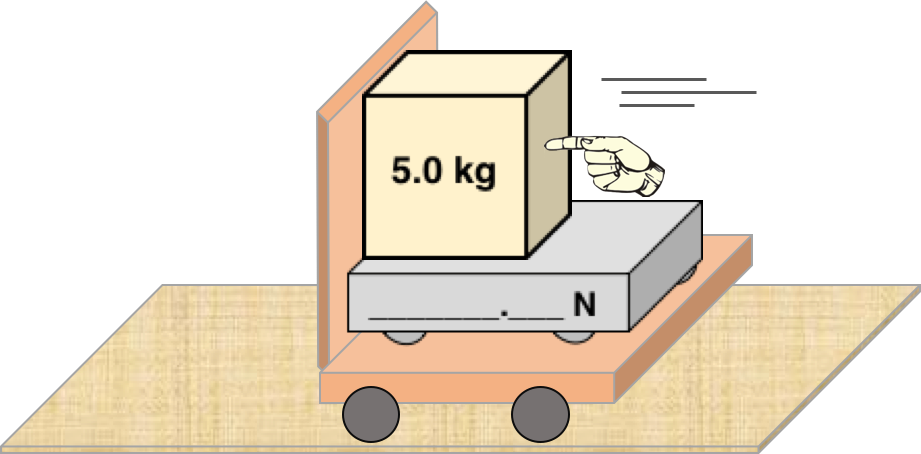Question 11
Consider the following situation and categorize it according to the relative strength of the normal force (or scale reading). Category options include:
1. The normal force is equal to the weight of the object.
2. The normal force is greater than (>)the weight of the object.
3. The normal force is less than (<) the weight of the object.
4. There is not enough information to decide.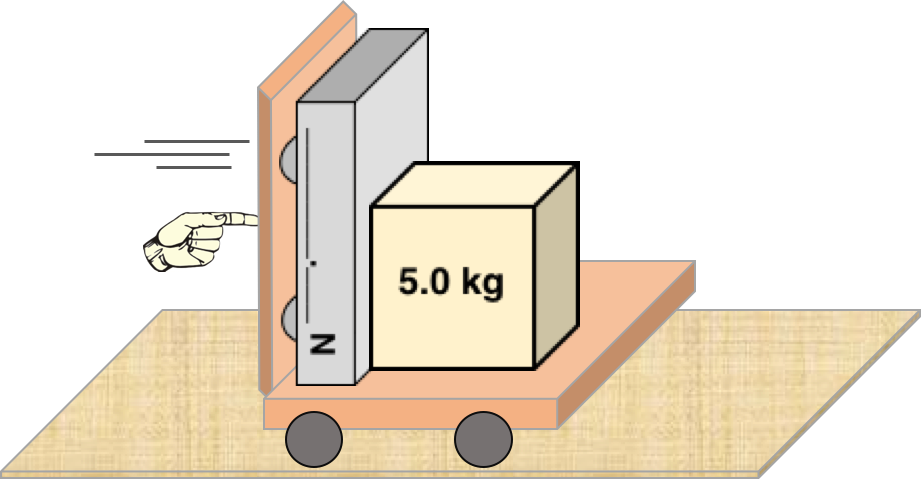Question 12
Consider the following situation and categorize it according to the relative strength of the normal force (or scale reading). Category options include:
1. The normal force is equal to the weight of the object.
2. The normal force is greater than (>)the weight of the object.
3. The normal force is less than (<) the weight of the object.
4. There is not enough information to decide.

A special thanks to Professor Brian Frank (@brianwfrank) for inspiring this idea.

View:  Questions in PDF Format

Visit: Concept Builder​Teacher Notes